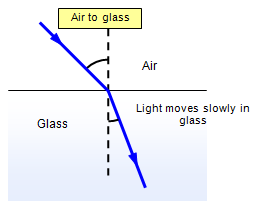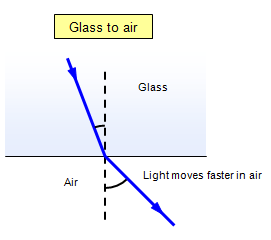# RefractionWhen light passes from air into a transparent and denser material such as glass, water or plastic it slows down.
If it meets the join between the materials at an angle then it bends – this is called refraction.
The light bends towards the normal line so that the angle of refraction is less than the angle of incidence. The amount of bending for a given angle of incidence depends on the material. It is greater for diamond than for glass and greater for glass than it is for water.

The list below shows the angles of refraction in various materials for an angle of incidence in air of 40o:
Water 29o a change of direction of 11o
Glass 25o a change of direction of 15o
Diamond 16o a change of direction of 24o

(Remember that we are always dealing with the angle between the beam of light and the normal line at right angles to the boundary between the two materials).This amount of bending is also related to the change of speed of the light. Light travels slower in diamond than it does in glass and slower in glass then it does in water.

Speed of light in air = 300 000 km/s Speed of light in glass = 200 000 km/s
Speed of light in water = 226 000 km/s
Speed of light in diamond = 125 000 km/s

When light travels from air into a denser material such as water or glass it slows down and bends towards the normal. When light travels into air from a denser material such as water or glass it speeds up and bends away from the normal.

If it were not for refraction, lenses would not work and optical instruments like cameras, telescopes, glasses, contact lenses, magnifying glasses and even your eyes would not work.

## The mathematics of refraction – Snell's Law

The amount of bending (refraction) depends on a property of the material known as its REFRACTIVE INDEX. This property connects the angle of incidence (i) with the angle of refraction (r). The law relating these two angles was discovered by Snell in 1621 and is known as Snell's Law.

Snells Law: Refractive index = sin i/sin r

The greater the refractive index the more the light refracts. Glass has a refractive index of 1.5, water 1.3 and diamond 2.42. This means that light will bend more when it hits a diamond than it will when it hits a piece of glass of the same shape. It is partly this that makes diamonds sparkle so much.

Example problem
A ray of light hits a glass block which has a refractive index of 1.5 at an angle of incidence of 30o.
Calculate the angle of refraction.

sin i/ sin r = 1.5 = sin 30/sin r    therefore sin r = 0.33    r =19.6o### schoolphysics Snell's Law interactive experiment

To see an interactive experiment to investigate Snell's law click on the animation link.

© Keith Gibbs 2013